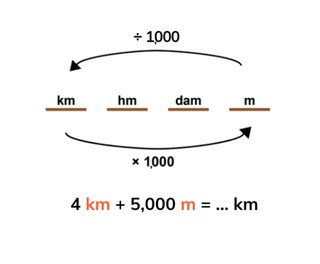Students learn to add meters and kilometers.

No account needed.8,000 schools use Gynzy92,000 teachers use Gynzy1,600,000 students use Gynzy

## General

Students learn how they can add meters and kilometers together one another.

## Standards

CCSS.Math.Content.4.MD.A.2

## Learning objective

Students will be able to add meters (m) and kilometers (km) together.

## Introduction

There are several numbers on the interactive whiteboard, for which the students must convert the kilometers to meters and vice versa.

## Instruction

Ask the students how many meters there are in one kilometer. Explain how you can add meters and kilometers to each other. Say that you must first convert the kilometers to meters, then you can add them up. For this you take three jumps to the right, so you multiply by 1000. Discuss several problems as a class. Next the students must complete an addition problem, for which they can choose from a few possible answers or give the answer by themselves. Then you explain that you can also convert to kilometers. You take three jumps to the left, so you divide by 1000. Have the students solve a few problems on their own. Then you discuss adding meters and kilometers in the form of a story problem. Have the students solve a story problem on their own.

To check whether students can add meters and kilometers, you can ask the following questions:
- How do you add meters and kilometers, when you must give the answer in kilometers?
- How do you add meters and kilometers when you must give the answer in meters?

## Quiz

Students first practice adding meters and kilometers with and without possible answers given. Afterwards they make a story problem, which they then must solve.

## Closing

You discuss again with the students how it is important to be able to add with meters and kilometers, because then you can determine how much something is in total. Check if they know that they must first convert to the same length measurement by multiplying or dividing by 1000. Show a story problem on the board that contains a few missing numbers. The students must add the meters and kilometers together. Then they can use the sum to calculate next missing number in the text.

## Teaching tips

When students have difficulty with adding meters and kilometers, you can give them a copy of the metric system, in which you only have meters and kilometers with an arrow for ÷1000 and an arrow for x1000. Explain that you must first convert. Then you can add the numbers together.

## Instruction materials

You may print out an image of the metric system.

### The online teaching platform for interactive whiteboards and displays in schools

• Save time building lessons

• Manage the classroom more efficiently

• Increase student engagement